Author: Oscar Cronquist Article last updated on December 28, 2020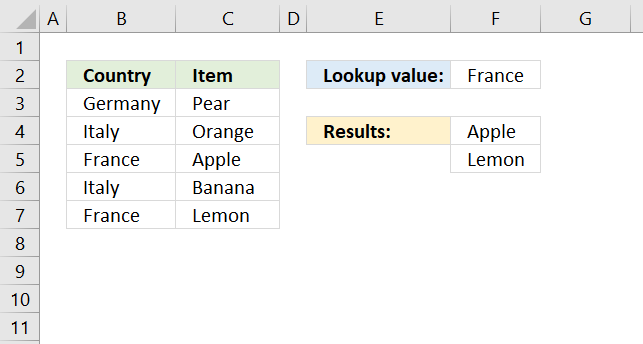The FILTER function lets you extract values/rows based on a condition or criteria. It is in the Lookup and reference category and is only available to Excel 365 subscribers.

The image above shows a regular formula in cell D3:

=FILTER(C3:C7, B3:B7=F2)

The formula above filters the data set in cell range C3:C7 based on the condition specified in cell F2. The output is an array and is returned to cell F4 and cells below as far as needed.

### Excel Function Syntax

FILTER(array, include, [if_empty])

### Arguments

 Argument Text array Required. Cell range or array. include Required. An array containing True or False that match the rows and columns of the array argument. [if_empty] Optional. The FILTER function returns #CALC! if the result is empty. [if_empty] is a value you define to avoid the #CALC! error.

### 1 Filter values based on a conditionThe Filter function filters values in cell range C3:C7 if a value in B3:B7 on the corresponding row equals "France" (Cell F2).

Formula in cell D3:

=FILTER(C3:C7, B3:B7=F2)

There is a formula for you that have an earlier Excel version than Excel 365: VLOOKUP - Return multiple values [vertically]

The FILTER function has three arguments, the second one is an array that evaluates to TRUE or FALSE or their numerical equivalents 1 or 0 (zero).

FILTER(array, include, [if_empty])

B3:B7=F2

The equal sign is a logical operator that evaluates if cell values in B3:B7 are equal to cell value in F2, one by one.

Note, the equal sign is not a case-sensitive. For example, "m" = "M" evaluates to True.

B3:B7=F2

becomes

{"Germany";"Italy";"France";"Italy";"France"}="France"

becomes

{"Germany"="France";"Italy"="France";"France"="France";"Italy"="France";"France"="France"}

and returns {FALSE; FALSE; TRUE; FALSE; TRUE}.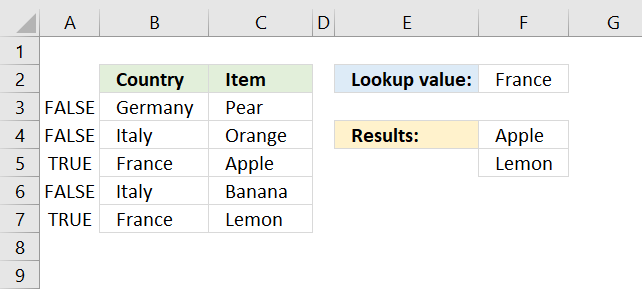The image above shows the array in column A, the values correspond to the values in column B, True is on the same row as France row 5 and 7. False is on all remaining rows.

The FILTER function uses this array containing boolean values (TRUE or False) to determine which values to return from column C. "Apple" and "Lemon" are on the same row as France row 5 and 7. The values are returned to cell F4 and cells below if needed.

The FILTER function may return an array and Microsoft calls this a dynamic array meaning the values are automatically entered to cells below without the need for an array formula.

#### 1.1 Why does the FILTER function return a #NAME? error?

First, make sure you correctly spelled the FILTER function. If the FILTER function still returns a #NAME? error you probably use an earlier Excel version and can't the FILTER function unless you upgrade to Excel 365.

There is a small formula you can use if you don't have access to the FILTER function, check it out here: VLOOKUP - Return multiple values [vertically]

#### 1.2 Is the FILTER function available for Excel versions 2003, 2007, 2010, 2013, 2016, and 2019?

No, only Excel 365 subscribers have it. However, I made a small formula that works fine, check it out here: VLOOKUP - Return multiple values [vertically]

#### 1.3 The FILTER function returns a #SPILL! error, why?

The FILTER function returns an array of values and tries to automatically use the appropriate cell range needed to show all values. If one or more cells are occupied with other values the FILTER  function returns #SPILL! error.

You have two options, delete or move the values that cause the error or deploy the FILTER function in another cell that has empty cells below.

#### 1.4 Why does the FILTER function return a #CALC! error?

The FILTER function returns a #CALC! error if the output result has no values, make sure you enter the third argument to define a value if dynamic array is empty, to avoid the #CALC! error.

#### 1.5 Can I use the FILTER function with an Excel Table and structured references?

Yes, you can. The FILTER function recalculates the output automatically if you add, edit or delete values in the Excel Table.

Note, the FILTER function does not care if the Excel Table is filtered or not, all matching values will be displayed hidden or not.

#### 1.6 Can the FILTER function handle error values?

No, the FILTER function stops working if there are error values in the second argument. An error in the first argument is passed on to the output array with other regular values.

#### 1.7 Is the FILTER function case sensitive?

No, the FILTER function is not case sensitive if you use the equal sign. Read the next section for how to make the FILTER function case sensitive.

### 2 Filter values based on a condition - case sensitive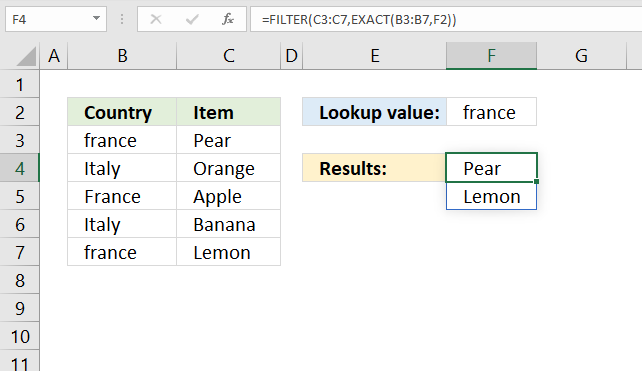The image above shows a formula in cell F4 that extracts values from column C if the corresponding values in column B match the value in cell F2.

=FILTER(C3:C7,EXACT(B3:B7,F2))

There is a formula for you that have an earlier Excel version than Excel 365: Case sensitive lookup and return multiple values

The EXACT function returns True if both arguments are exactly the same considering upper and lower letters as well.

EXACT(text1, text2)

The EXACT function allows you to use a cell range in the first argument and the result is an array that matches the size of the first argument as long as the second argument is a single value.

EXACT(B3:B7,F2)

becomes

EXACT({"france"; "Italy"; "France"; "Italy"; "france"}, "france")

and returns {TRUE; FALSE; FALSE; FALSE; TRUE}.

FILTER(C3:C7,EXACT(B3:B7,F2))

becomes

FILTER(C3:C7,{TRUE; FALSE; FALSE; FALSE; TRUE})

becomes

FILTER({"Pear"; "Orange"; "Apple"; "Banana"; "Lemon"}, {TRUE; FALSE; FALSE; FALSE; TRUE})

and returns {"Pear"; "Lemon"} in cell range F4:F5.

### 3 Filter values if not equal to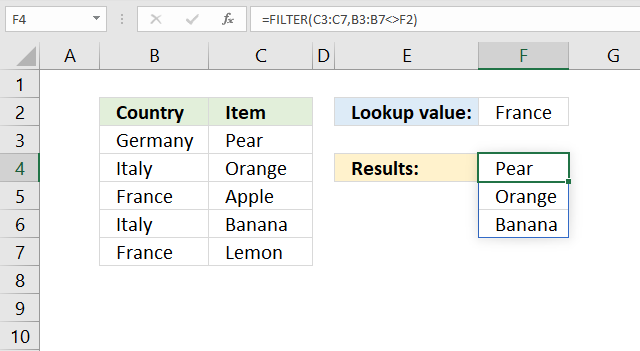The image above demonstrates the FILTER function in cell F4, it returns all values from column C if the corresponding values in column B are not equal to the value in cell F2.

=FILTER(C3:C7,B3:B7<>F2)

The less than and larger than characters combined means "not equal to".

B3:B7<>F2

becomes

{"Germany"; "Italy"; "France"; "Italy"; "France"}<>"France"

and returns {TRUE; TRUE; FALSE; TRUE; FALSE}.

FILTER(C3:C7,B3:B7<>F2)

becomes

FILTER({"Pear";"Orange";"Apple";"Banana";"Lemon"}, {TRUE; TRUE; FALSE; TRUE; FALSE})

and returns {"Pear"; "Orange"; "Banana"} in cell range F4:F6.

### 4 Filter values smaller than a condition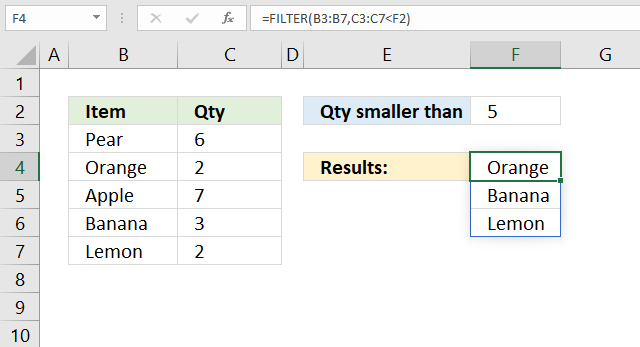The image above shows a formula in cell F4 that extracts values from column B if the corresponding value in column C is less than the number in cell F2.

=FILTER(B3:B7,C3:C7<F2)

The less than character < is a logical operator that checks if a number is smaller than another number. In this demonstration, we will apply this to a cell range C3:C7. The logical expression is:

C3:C7<F2

becomes

{6;2;7;3;2}<5

and returns {FALSE; TRUE; FALSE; TRUE; TRUE}.

FILTER(B3:B7, C3:C7<F2)

becomes

FILTER({"Pear"; "Orange"; "Apple"; "Banana"; "Lemon"}, {FALSE; TRUE; FALSE; TRUE; TRUE})

and returns {"Orange"; "Banana"; "Lemon"}.

### 5 Filter values if larger than the given condition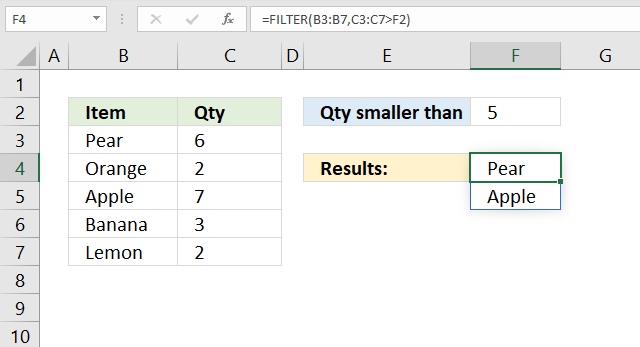Formula in cell F4:

=FILTER(B3:B7,C3:C7>F2)

The larger than character > is a logical operator that evaluates to True if a number is larger than another number otherwise False. In this demonstration, we will apply this to a cell range C3:C7. The logical expression is:

C3:C7>F2

becomes

{6;2;7;3;2}>5

and returns {TRUE; FALSE; TRUE; FALSE; FALSE}.

FILTER(B3:B7, C3:C7>F2)

becomes

FILTER({"Pear";"Orange";"Apple";"Banana";"Lemon"}, {TRUE; FALSE; TRUE; FALSE; FALSE})

and returns {"Pear"; "Apple"}.

### 6 Filter values if cell contains given string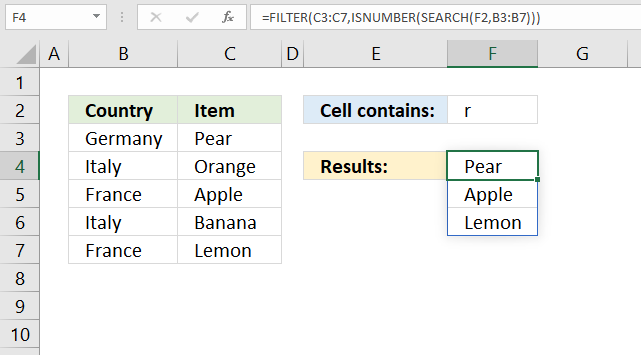The image above shows a formula in cell F4 that extracts values from column C if the corresponding value in column B contains the given string in cell F2.

Formula in cell F4:

=FILTER(C3:C7,ISNUMBER(SEARCH(F2,B3:B7)))

The formula in cell F4 returns "Pear", "Apple" and "Lemon" because the corresponding values in column B "Germany", "France" and "France" contain string "r".

#### Step 1 - Identify cells containing string

The SEARCH function has these arguments: SEARCH(find_text,within_text, [start_num])

It returns a number representing the character position of the found string, an error is returned if not found. The SEARCH function does not perform a case sensitive search, use the FIND function for that.

SEARCH(F2,B3:B7))

becomes

SEARCH("r",{"Germany"; "Italy"; "France"; "Italy"; "France"}))

and returns {3; #VALUE!; 2; #VALUE!; 2}.

#### Step 2 - Replace errors

The FILTER function can't handle errors in the second argument include. FILTER(array, include, [if_empty])

ISNUMBER(SEARCH(F2,B3:B7))

becomes

ISNUMBER({3; #VALUE!; 2; #VALUE!; 2})

and returns {TRUE; FALSE; TRUE; FALSE; TRUE}.

#### Step 3 - Filter array

FILTER(C3:C7,ISNUMBER(SEARCH(F2,B3:B7)))

becomes

FILTER(C3:C7, {TRUE; FALSE; TRUE; FALSE; TRUE})

becomes

FILTER({"Pear"; "Orange"; "Apple"; "Banana"; "Lemon"}, {TRUE; FALSE; TRUE; FALSE; TRUE})

and returns {"Pear"; "Apple"; "Lemon"}.

### 7 Filter values if cell doesn't contain the given string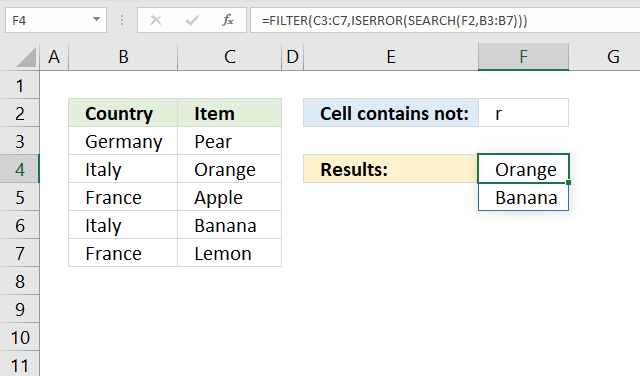The image above shows a formula in cell F4 that extracts values from column C if the corresponding value in column B does not contain the given string in cell F2.

Formula in cell F4:

=FILTER(C3:C7,ISERROR(SEARCH(F2,B3:B7)))

The formula in cell F4 returns "Orange" and "Banana" because the corresponding values in column B "Italy" and "Italy" do not contain string "r".

#### Step 1 - Identify cells that contain the string

The SEARCH function has these arguments: SEARCH(find_text,within_text, [start_num])

It returns a number representing the character position of the found string, an error is returned if not found. The SEARCH function does not perform a case sensitive search, use the FIND function for that.

SEARCH(F2,B3:B7))

becomes

SEARCH("r",{"Germany"; "Italy"; "France"; "Italy"; "France"}))

and returns {3; #VALUE!; 2; #VALUE!; 2}.

#### Step 2 - Identify errors

The FILTER function can't handle errors in the second argument include. FILTER(array, include, [if_empty])

ISERROR(SEARCH(F2,B3:B7))

becomes

ISNUMBER({3; #VALUE!; 2; #VALUE!; 2})

and returns {FALSE; TRUE; FALSE; TRUE; FALSE}.

#### Step 3 - Filter array

FILTER(C3:C7, ISERROR(SEARCH(F2,B3:B7)))

becomes

FILTER(C3:C7, {FALSE; TRUE; FALSE; TRUE; FALSE})

becomes

FILTER({"Pear"; "Orange"; "Apple"; "Banana"; "Lemon"}, {FALSE; TRUE; FALSE; TRUE; FALSE})

and returns {"Orange"; "Banana"} in cell range F4:F5.

### 8 Filter values that begin with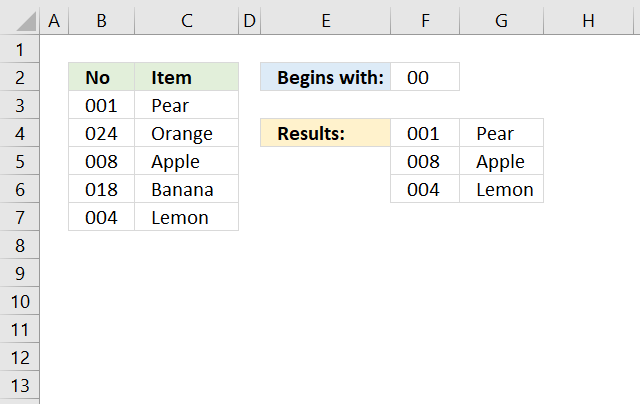The formula above in cell F4 extracts values from column C if the corresponding value in column B begins with the given string in cell F2.

Formula in cell F4:

=FILTER(B3:C7,LEFT(B3:B7,LEN(F2))=F2)

The formula in cell F4 returns all rows from cell range B3:C7 that begins with the string specified in cell F2.

#### Step 1 - Calculate character length of value in cell F2

The LEN function counts the characters in a cell or string.

LEN(F2)

becomes

LEN("00")

and returns 2.

#### Step 2 - Extract characters

The LEFT function returns a specific number of characters from a cell value or string starting from the left. LEFT(text,[num_chars])

LEFT(B3:B7,LEN(F2))

becomes

LEFT(B3:B7,2)

becomes

LEFT({"001"; "024"; "008"; "018"; "004"}, 2)

and returns {"00";"02";"00";"01";"00"}.

#### Step 3 - Compare with value in cell F2

The equal sign lets you compare values (not case sensitive), it returns a boolean value True or False.

LEFT(B3:B7,LEN(F2))=F2

becomes

{"00";"02";"00";"01";"00"}="00"

and returns

{TRUE; FALSE; TRUE; FALSE; TRUE}.

#### Step 4 - Filter values

FILTER(B3:C7,LEFT(B3:B7,LEN(F2))=F2)

becomes

FILTER(B3:C7,{TRUE; FALSE; TRUE; FALSE; TRUE})

becomes

FILTER({"001", "Pear"; "024", "Orange"; "008", "Apple"; "018", "Banana"; "004", "Lemon"},{TRUE; FALSE; TRUE; FALSE; TRUE})

and returns

{"001","Pear";"008","Apple";"004","Lemon"} in cell range F4:G7.

### 9 Filter values that end with a specific string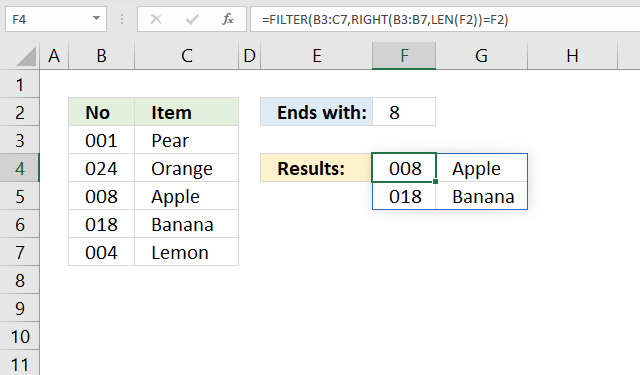The formula above in cell F4 extracts values from column C if the corresponding value in column B ends with the given string in cell F2.

Formula in cell F4:

=FILTER(B3:C7, RIGHT(B3:B7, LEN(F2))=F2)

The formula in cell F4 returns all rows from cell range B3:C7 that end with 8 in column B.

#### Step 1 - Calculate character length of the value in cell F2

The LEN function counts the characters in a cell or string.

LEN(F2)

becomes

LEN("8")

and returns 1.

#### Step 2 - Extract characters

The RIGHT function returns a specific number of characters from a cell value or string starting from the left. LEFT(text,[num_chars])

LEFT(B3:B7,LEN(F2))

becomes

LEFT(B3:B7,1)

becomes

LEFT({"001"; "024"; "008"; "018"; "004"}, 1)

and returns {"1";"4";"8";"8";"4"}.

#### Step 3 - Compare with value in cell F2

The equal sign lets you compare values (not case sensitive), it returns a boolean value True or False.

RIGHT(B3:B7,LEN(F2))=F2

becomes

{"1";"4";"8";"8";"4"}="8"

and returns

{FALSE; FALSE; TRUE; TRUE; FALSE}.

#### Step 4 - Filter values

FILTER(B3:C7,LEFT(B3:B7,LEN(F2))=F2)

becomes

FILTER(B3:C7, {FALSE; FALSE; TRUE; TRUE; FALSE})

becomes

FILTER({"001", "Pear"; "024", "Orange"; "008", "Apple"; "018", "Banana"; "004", "Lemon"}, {FALSE; FALSE; TRUE; TRUE; FALSE})

and returns

{"008", "Apple"; "018", "Banana"} in cell range F4:G5.

### 10 Filter values based on a condition sorted A to Z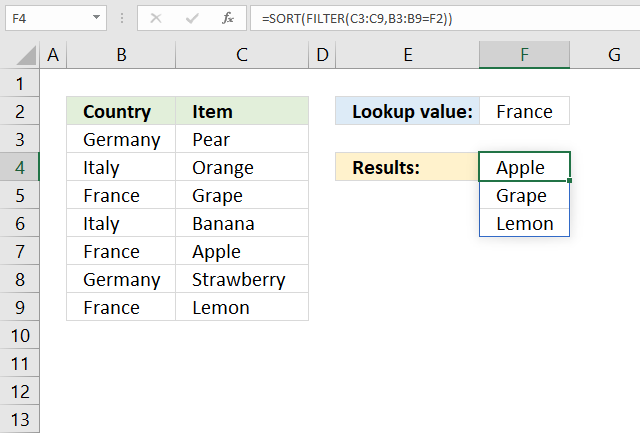Formula in cell F4:

=SORT(FILTER(C3:C9,B3:B9=F2))

The formula in cell F4 extracts values from column B based on a condition specified in cell F2, If the condition matches a value in column C the corresponding value from column B is returned. The array is sorted from A to Z.

#### Step 1 - Logical expression

The equal sign allows you to compare the value in cell F2 to values in cell range B3:B9. The result is an array containing boolean values True or False.

B3:B9=F2

becomes

{"Germany"; "Italy"; "France"; "Italy"; "France"; "Germany"; "France"}="France"

and returns

{FALSE; FALSE; TRUE; FALSE; TRUE; FALSE; TRUE}

#### Step 2 - Filter values

The array containing boolean values determine which values in cell range C3:C9 to filter.

FILTER(C3:C9,B3:B9=F2)

becomes

FILTER(C3:C9,{FALSE; FALSE; TRUE; FALSE; TRUE; FALSE; TRUE})

becomes

FILTER({"Pear"; "Orange"; "Grape"; "Banana"; "Apple"; "Strawberry"; "Lemon"}, {FALSE; FALSE; TRUE; FALSE; TRUE; FALSE; TRUE})

and returns

{"Grape"; "Apple"; "Lemon"}.

#### Step 3 - Sort values

The SORT function is available for Excel 365 users and has the following arguments: SORT(array,[sort_index],[sort_order],[by_col])

It lets you sort an array from A to Z if you leave the remaining arguments, they are optional anyway.

SORT(FILTER(C3:C9,B3:B9=F2))

becomes

SORT({"Grape"; "Apple"; "Lemon"})

and returns

{"Apple"; "Grape"; "Lemon"}

in cell range F4:F6.

Note, the following formula sorts from Z to A:

=SORT(FILTER(C3:C9, B3:B9=F2),,-1)

### 11 Filter values based on criteria applied to a column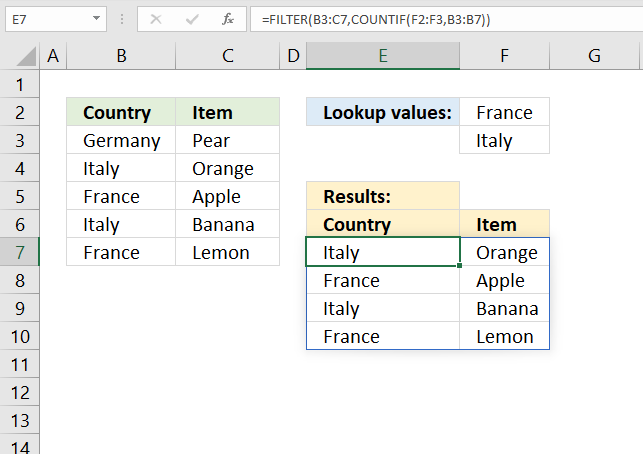The formula in cell E7 filters the values in cell range B3:C7 based on two values specified in cell range F2:F3.

If any of the two values match a value in column B the corresponding value from column C is returned as well as the matching value.

Formula in cell E7:

=FILTER(B3:C7, COUNTIF(F2:F3, B3:B7))

The COUNTIF function counts values based on a condition or criteria, here is how it is done. COUNTIF(range, criteria)

COUNTIF(F2:F3, B3:B7)

becomes

COUNTIF({"France"; "Italy"}, {"Germany"; "Italy"; "France"; "Italy"; "France"})

and returns

{0; 1; 1; 1; 1}. 0 (zero) is the equivalent to False and every other value positive or negative evaluates to True.

FILTER(B3:C7, {0; 1; 1; 1; 1})

becomes

FILTER({"Germany", "Pear"; "Italy", "Orange"; "France", "Apple"; "Italy", "Banana"; "France", "Lemon"}, {0; 1; 1; 1; 1})

and returns

{"Italy", "Orange"; "France", "Apple"; "Italy", "Banana"; "France", "Lemon"}.

### 12 Filter values based on one condition per column - AND logic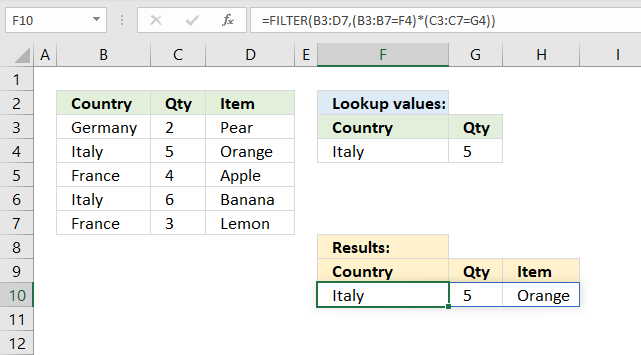The formula in cell F10 extracts rows from cell range B3:D7 if two conditions are met, specified in cell F4 and G4. The first condition is compared to column B and the second condition is compared to column C.

A row is extracted if both conditions are met on the same row.

Formula in cell F7:

=FILTER(B3:D7, (B3:B7=F4)*(C3:C7=G4))

The first logical expression is B3:B7=F4.

B3:B7=F4

becomes

{"Germany";"Italy";"France";"Italy";"France"}="Italy"

and returns

{FALSE; TRUE; FALSE; TRUE; FALSE}

The second logical expression is {2; 5; 4; 6; 3}=5.

{2; 5; 4; 6; 3}=5

returns

{FALSE; TRUE; FALSE; FALSE; FALSE}

To apply AND logic we must multiply the arrays using the asterisk character. The parentheses allow us to control the calculations, we want to calculate the expressions inside the parentheses before we multiple the arrays.

(B3:B7=F4)*(C3:C7=G4)

becomes

({FALSE; TRUE; FALSE; TRUE; FALSE})*({FALSE; TRUE; FALSE; FALSE; FALSE})

and returns

{0; 1; 0; 0; 0}.

Boolean values are automatically converted to their numerical equivalents when we calculate arithmetic operations.

True * True equals 1

True * False equals 0 (zero)

False * True equals 0 (zero)

False * False equals 0 (zero)

FILTER(B3:D7, (B3:B7=F4)*(C3:C7=G4))

becomes

FILTER(B3:D7, {0; 1; 0; 0; 0})

becomes

FILTER({"Germany", 2, "Pear"; "Italy", 5, "Orange"; "France", 4, "Apple"; "Italy", 6, "Banana"; "France", 5, "Lemon"}, {0; 1; 0; 0; 0})

and returns {"Italy", 5, "Orange"}.

### 13 Filter values based on one condition per column - OR logic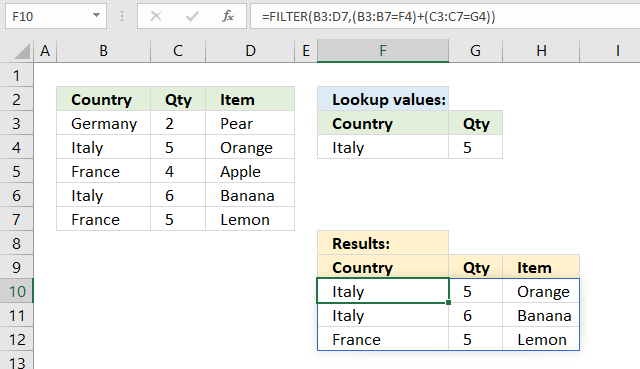The formula in cell F10 extracts rows from cell range B3:D7 if any of the two conditions are met, specified in cell F4 and G4. The first condition is compared to column B and the second condition is compared to column C.

A row is extracted if any of the conditions are met on the same row.

Formula in cell F7:

=FILTER(B3:D7, (B3:B7=F4)+(C3:C7=G4))

The first logical expression is B3:B7=F4.

B3:B7=F4

becomes

{"Germany";"Italy";"France";"Italy";"France"}="Italy"

and returns

{FALSE; TRUE; FALSE; TRUE; FALSE}

The second logical expression is {2; 5; 4; 6; 3}=5.

{2; 5; 4; 6; 5}=5

returns

{FALSE; TRUE; FALSE; FALSE; TRUE}

(B3:B7=F4)+(C3:C7=G4)

becomes

({FALSE; TRUE; FALSE; TRUE; FALSE}) + ({FALSE; TRUE; FALSE; FALSE; TRUE})

and returns

{0; 2; 0; 1; 1}

True + True equals 2 (True)

True + False equals 1 (True)

False + True equals 1 (True)

False + False equals 0 (False)

FILTER(B3:D7, (B3:B7=F4)+(C3:C7=G4))

becomes

FILTER({"Germany", 2, "Pear"; "Italy", 5, "Orange"; "France", 4, "Apple"; "Italy", 6, "Banana"; "France", 5, "Lemon"}, {0; 2; 0; 1; 1})

and returns

{"Italy", 5, "Orange"; "Italy", 6, "Banana"; "France", 5, "Lemon"}.

### 14 #CALC! error returns [if_empty] argument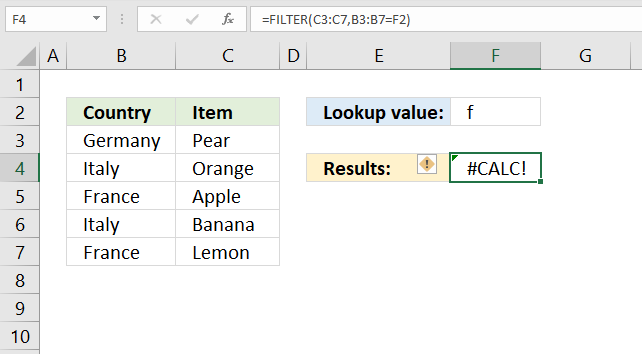The FILTER function in cell F4 returns a #CALC! error. The value in cell F2 is not found in any of the cells in B3:B7.

=FILTER(C3:C7,B3:B7=F2)

The FILTER function returns #CALC! error instead of an empty array. The third argument allows you to customize what to return if the FILTER function returns nothing, see below.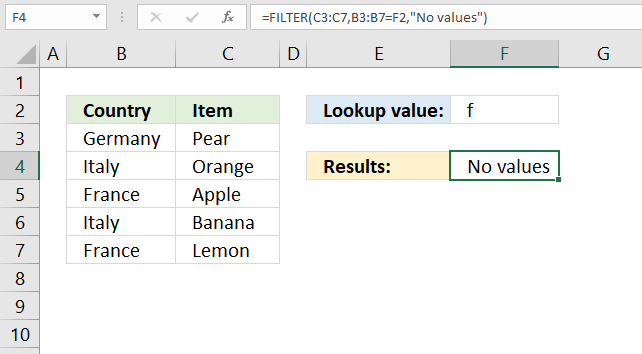=FILTER(C3:C7,B3:B7=F2,"No values")